# Contract forecast

## Overview

• Contract forecast
• Calculation logic behind the forecasts
• Difference between real case and worst case scenarios
• Consideration of target and actual costs
• Deduction of discounts, cost allocations, insurances and cash discounts

Alasco distinguishes between contract and project forecasts. Both types can be presented in the real case and the worst case scenarios. Both the project and contract forecasts are based on costs.

## Contract forecast

The contract forecast is used to make quick statements about a single contract. The contract forecast is used to calculate the project forecast once the contract unit is "Fully Awarded" or "Finalized", as described above.

You will find the contract forecast in the cost controlling as the columns "Contract Cost Forecast (Real Case)" and "Contract Cost Forecast (Worst Case)".

You can also display the deviation from the current budget in absolute values and percentages against the real and worst case scenarios of the contract forecast with the columns "Contract Budget Deviation Real/Worst Case" and "Contract Budget Deviation Real/Worst Case (%)".

## Calculation of the contract forecast

The contract forecast is calculated by adding or subtracting all relevant values of a contract. Which values are added or subtracted as part of the contract forecast can be freely configured for the real and worst case scenarios by an admin in the master data under the menu item "Forecast settings". These settings apply to the entire Alasco account.

## Real case actual costs example

Here you can see an example of the structure of a real case study.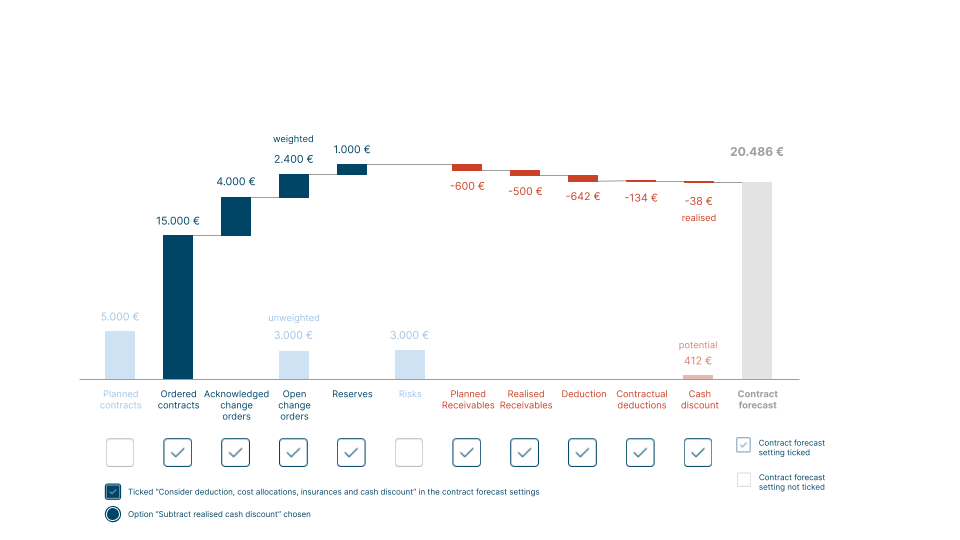## Worse case actual costs example

Here you can see an example of the structure of a worst case study.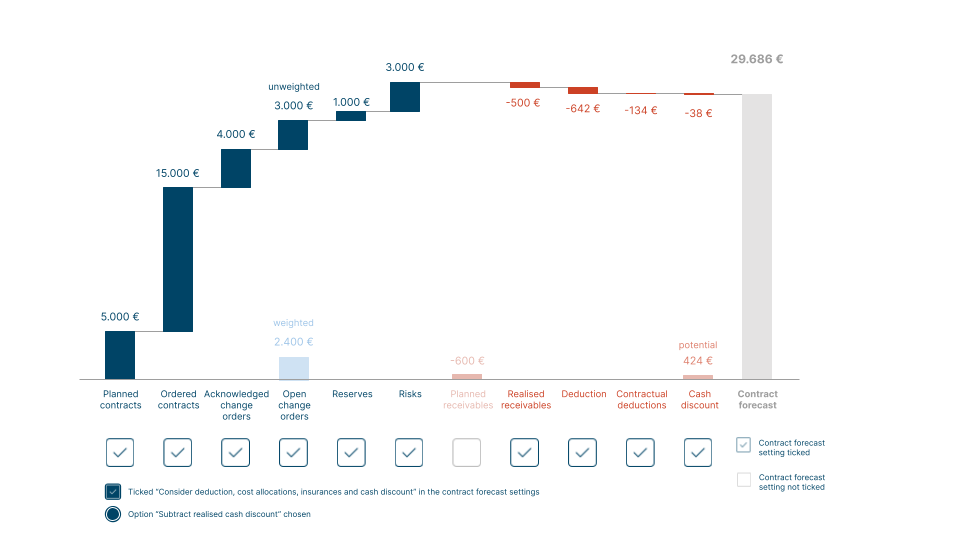## Actual costs settings example

These are the sample settings in the "Forecast settings" used for the above example.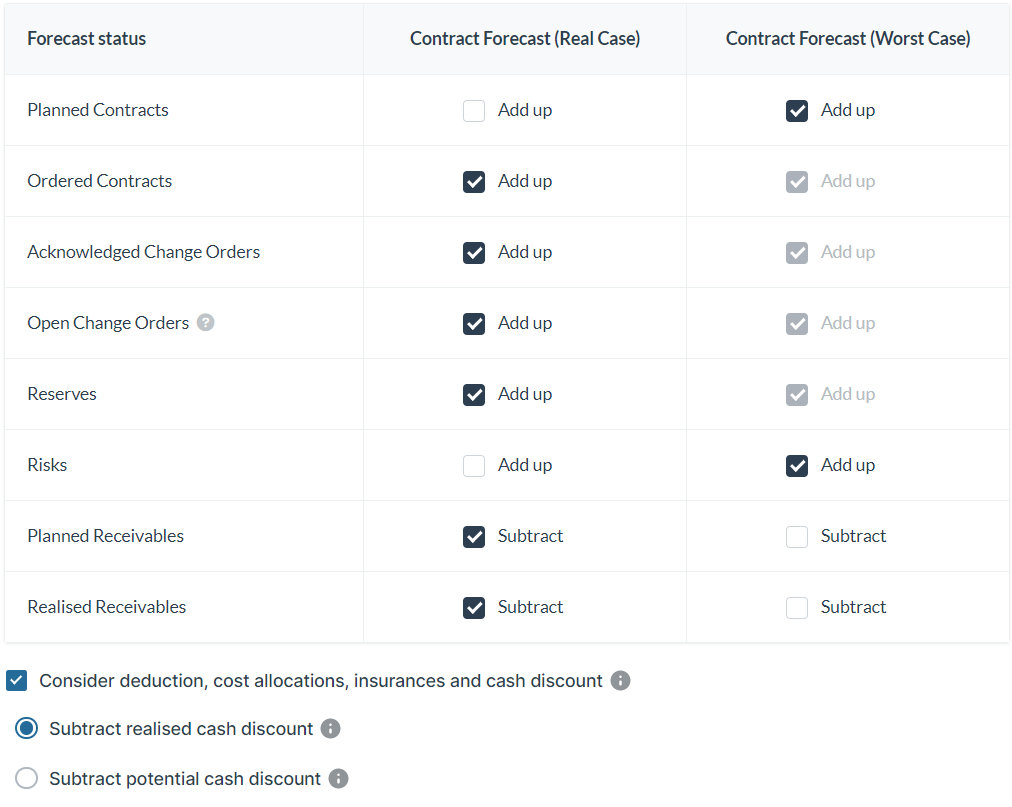Depending on the status of a contract, either the maximum of target vs actual costs or only the actual costs are used to calculate the contract forecast.

## Contract with the status Draft, Ordered or Partially Completed

As long as a contract has the status "Draft", "Ordered", or "Partially Completed", it depends on whether the sum of the target costs or the actual costs is higher.

The target costs come from the contract volume; the actual costs correspond to the paid invoices. Detailed lists for both target and actual costs can be found below.

Contract forecast (real case) = maximum of target costs or actual costs (real case)

Contract forecast (worst case) = maximum of target costs or actual costs (worst case)

## Contract with the status Completed

As soon as a contract has the status "Completed", the contract forecast corresponds to the actual costs, i.e. the approved invoices.

Contract forecast (real case) = actual costs (real case)

Contract forecast (worst case) = actual costs (worst case)

## Target costs

Target costs include the following data from a contract unit or contract:

• Draft contracts
• Main contracts
• Acknowledged change orders
• Open change orders
• Reserves
• Risks
• Planned receivables
• Realized receivables

In the master data, under the menu item "Forecast settings", an admin can define the individual items that should be added to the contract forecast for the real and worst case scenarios. Receivables can be subtracted.#### Note:

Please note that in Alasco all target and actual costs included in the real case forecast must also be included in the worst case forecast.

Conversely, all costs deducted in the worst case forecast must also be deducted in the real case forecast. The respective checkbox is greyed-out and can only be changed once the other checkmark has been removed from the respective forecast.

## Actual costs

Actual costs include the following data of a contract:

• Approved invoices
• Contractual retentions (general and defects liability retentions)
• Other retentions
• Planned receivables
• Realized receivables

In the master data, under the menu item "Forecast settings", an admin can define the individual items that should be added to the contract forecast for the real and worst case scenarios. Receivables can be subtracted.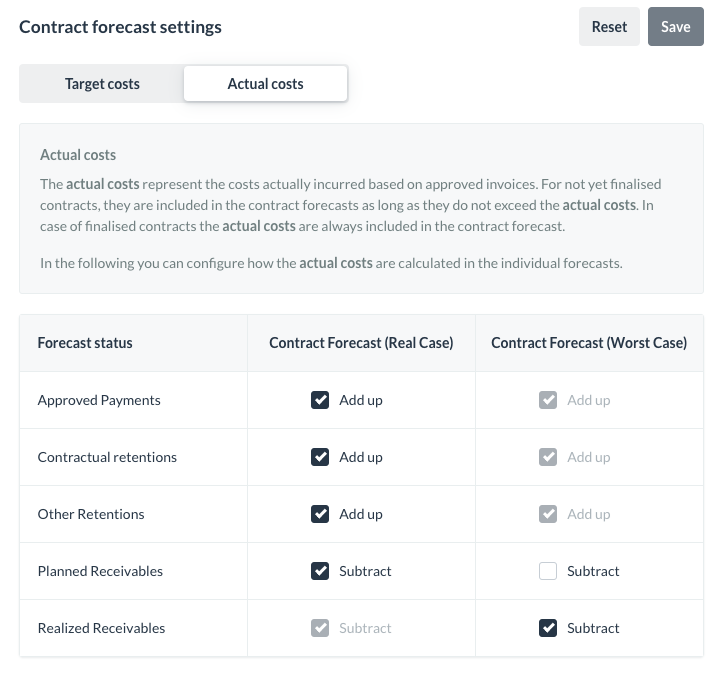#### Note:

Please note that in Alasco all target and actual costs included in the real case forecast must also be included in the worst case forecast.

Conversely, all costs deducted in the worst case forecast must also be deducted in the real case forecast. The respective checkbox is greyed-out and can only be changed once the other checkmark has been removed from the respective forecast.

## Consideration of contract terms and cash discount

In addition to the calculation logic of the forecasts mentioned above, you have the option to decide whether contract terms, such as deductions, cost allocations, insurances or cash discounts, are considered in the contract forecast.

If you activate this option in the forecast settings of the master data, the deductions for each contract are deducted from the target costs. No distinction is made between the real and worst case forecasts. The contract terms are calculated identically in both scenarios.

The actual costs are not affected, as the contract terms were already considered during invoice verification.

In principle, the contract terms, provided they are taken into account in the target costs, are subtracted as follows:

(Target contracts + main contracts + acknowledged change orders + open change orders) - contract terms

The subtraction of deductions, cost allocations and insurances is calculated the same way during the invoice verification. For the cash discount, you can define whether you want to subtract only the already realised cash discount or the total potential cash discount in the contract forecast.

## Subtract realised cash discount

If you select the option "Subtract realised cash discount", which is set as default, the cash discount actually taken from all paid invoices of a contract will be subtracted from the contract forecast. The cash discount amount corresponds to the amount entered during the workflow.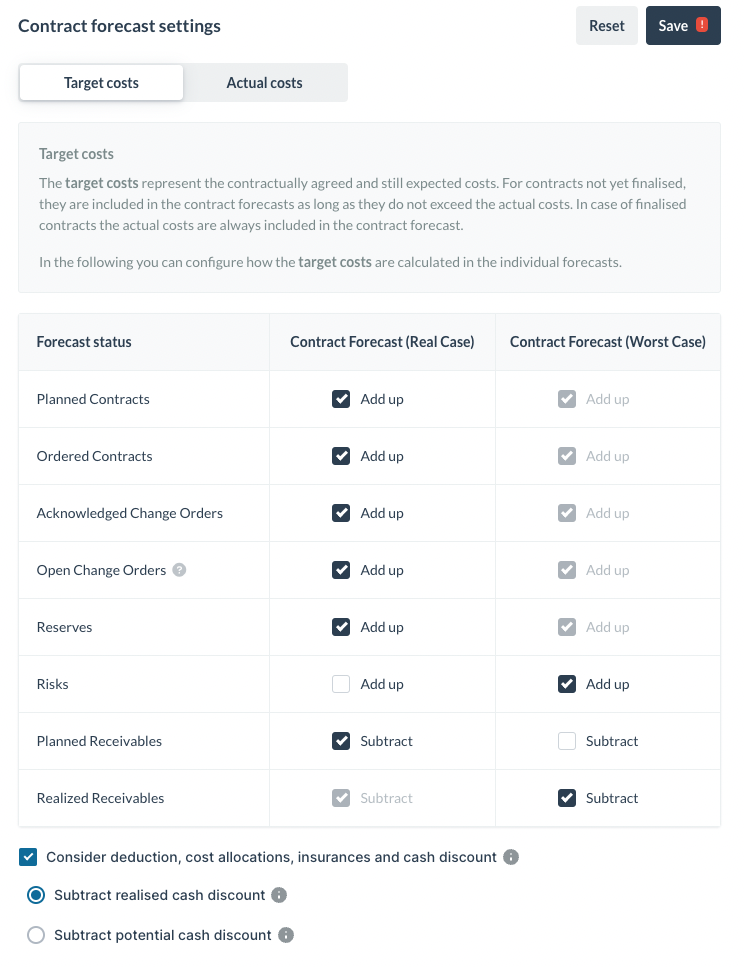#### Note:

In the last workflow step, "Pay invoice", you have the option to pay the "Approved amount less cash discount" or you can enter a different payment amount in the field "Other amount". This could be the case, for example, due to rounding differences.

If this deviating payment amount is within a tolerance of +- 5 cents of the released amount minus cash discount, the cash discount is considered as a "realized cash discount". Otherwise, the invoice is evaluated as if no cash discount was applied.

## Subtract potential cash discount

If you have selected the option "Subtract potential cash discount", the maximum possible cash discount amount from all contracts is subtracted from the contract forecast. Therefore, you can see the minimum amount you will have to pay if you approve and pay each invoice within the cash discount period.

As soon as an invoice is not paid within the cash discount period, the contract forecast is corrected by the corresponding unrealised cash discount.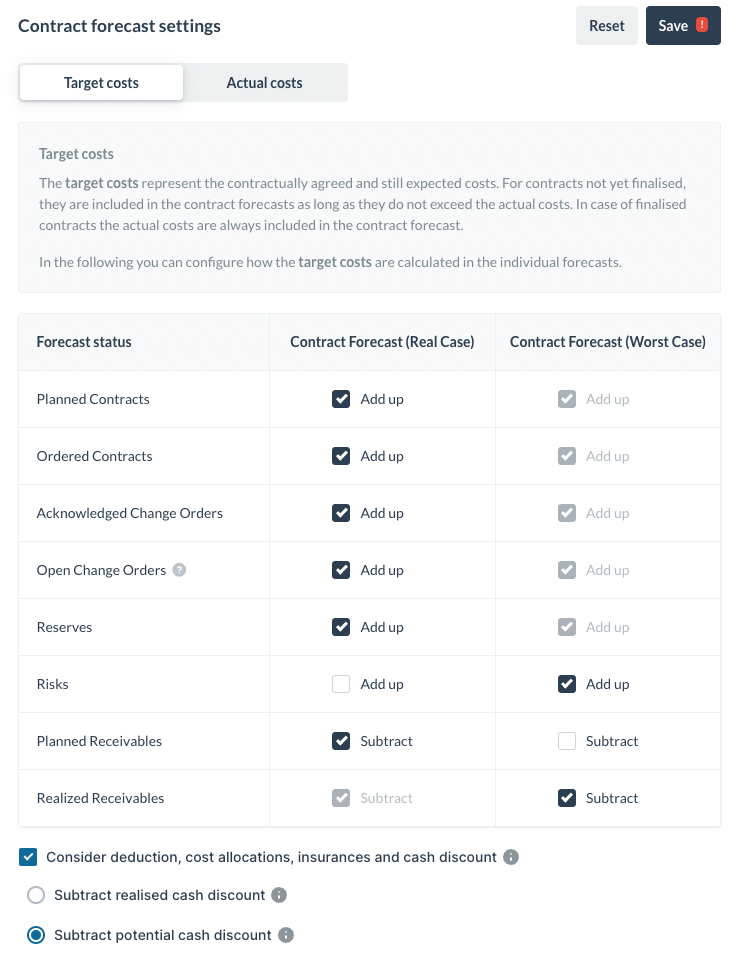#### Note:

Please note that these settings only apply to standard and multipart contracts. For recurring invoicing contracts, the forecast is calculated without considering contract terms and cash discounts.

LEGAL